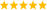# LabVIEW

cancel
Showing results for
Did you mean:

Solved!
Go to solution
Highlighted

## Calculations with long equations

Hello Everyone,

I am getting data from several sensors and I want to do calculations with them via Labview. The equations are long. Whats the procedure or method to do this?

Regards,
Alexis Koulidis

Message 1 of 17
(587 Views)
Highlighted

## Re: Calculations with long equations

Just implement the equations using LabVIEW code. Length is irrelevant.

If you tell us a little more about the problem, maybe we can give more specific advice. What does the formula do? How many inputs does it have?LabVIEW Champion. It all comes together inGCentralWhat does "Engineering Redefined" mean??
Message 2 of 17
(568 Views)
Highlighted

## Re: Calculations with long equations

Hello,

Thank you very much for your reply. I am getting data from 4 sensors, and I want calculate an other parameter that combines the sensors data with some constants.

Initially I was thinking to do with several Numeric and to each part of the addition, subtract or division separate, but I want to do all the calculations in once..If it is possible

I am giving an example of the formula: X,Y,Z and C are my input data from the sensors and 120,12,50 and 30 are constants

A = (120*pi*X)/(12*Y) + (Z*50)/(C+30)

Message 3 of 17
(557 Views)
Highlighted

## Re: Calculations with long equations

Personally, I find the Formula Node easier to read equations from (and therefore implement).  Others, like Altenbach, will go with the "pure" LabVIEW code (wire up all of the functions).Message 4 of 17
(549 Views)
Highlighted
Solution
Accepted by topic author Alexis_Koul

## Re: Calculations with long equations

@crossrulz wrote:

Personally, I find the Formula Node easier to read equations from (and therefore implement).  Others, like Altenbach, will go with the "pure" LabVIEW code (wire up all of the functions).Excellent.  A solution for everyone's tastes.  🙂

Bill(Mid-Level minion.)
My support system ensures that I don't look totally incompetent.
Proud to say that I've progressed beyond knowing just enough to be dangerous. I now know enough to know that I have no clue about anything at all.
Humble author of the CLAD Nugget.
Message 5 of 17
(535 Views)
Highlighted
Solution
Accepted by topic author Alexis_Koul

## Re: Calculations with long equations

Your solution looks excellent. One last question if I may. In your VI you put the X,Y,Z and C that you can put the values. In my case, I will just connect my data output correct with the numeric?

-Alexis

Message 6 of 17
(441 Views)
Highlighted
Solution
Accepted by topic author Alexis_Koul

## Re: Calculations with long equations

Though, a quick test show the formula node is noticably slower, so depending on your requirements it might be needed to use the nodes.

"Only dead fish swim downstream" - "My life for Kudos!" - "Dumb people repeat old mistakes - smart ones create new ones."Message 7 of 17
(422 Views)
Highlighted

## Re: Calculations with long equations

@Yamaeda wrote:

Though, a quick test show the formula node is noticably slower, so depending on your requirements it might be needed to use the nodes.

I'd like to see your benchmark and results.  I know the formula node will eliminate parallelism, but I have trouble believing it will be "noticeable" on a small equation like this.

Message 8 of 17
(414 Views)
Highlighted

## Re: Calculations with long equations

@Alexis_Koul wrote:

Your solution looks excellent. One last question if I may. In your VI you put the X,Y,Z and C that you can put the values. In my case, I will just connect my data output correct with the numeric?

I would turn what I showed you into a VI on its own with the controls and indicator connected on the Connector Pane.  This small block is then easily testable and reusable.  Your main VI will then just call this equation VI, wiring up the values to the right inputs.

Message 9 of 17
(413 Views)
Highlighted
Solution
Accepted by topic author Alexis_Koul

## Re: Calculations with long equations10M loops yields ~700 vs ~100ms (formula node in other case). So no, it's not noticable in a single run. But as i said, if the requirements are such that'll run many times in a tight loop, a factor of 8 is noticable. 🙂